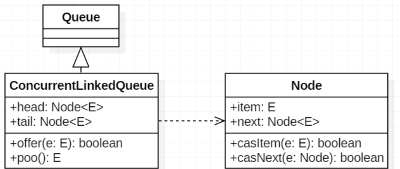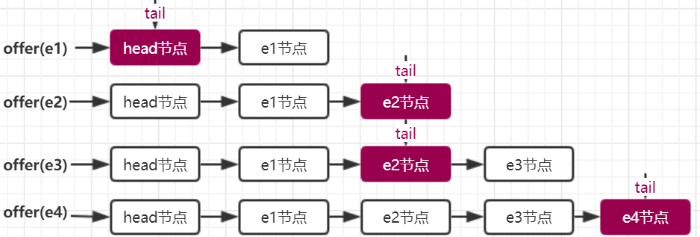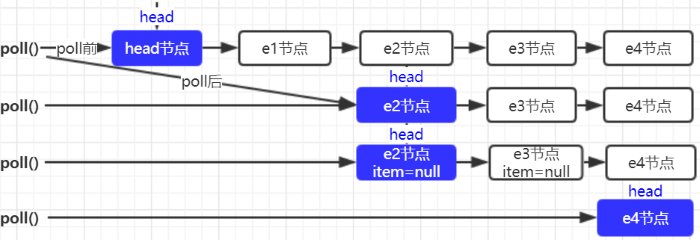• ConcurrentLinkedQueue 先进先出(FIFO)单向队列
• ConcurrentLinkedDeque 双向队列

## 1. 数据结构private transient volatile Node<E> head;
private transient volatile Node<E> tail;

private static class Node<E> {
volatile E item;
volatile Node<E> next;
}


public ConcurrentLinkedQueue() {
head = tail = new Node<E>(null);
}


// 遇到哨兵节点，从 head 开始遍历
final Node<E> succ(Node<E> p) {
Node<E> next = p.next;
return (p == next) ? head : next;
}


## 2. 入队 offerpublic boolean offer(E e) {
checkNotNull(e);
final Node<E> newNode = new Node<E>(e);

for (Node<E> t = tail, p = t;;) {
Node<E> q = p.next;
// 1. p is last node
if (q == null) {
// 1.1 通过自旋保证节点一定添加到数据链中
if (p.casNext(null, newNode)) {
// 1.2 p代表当前结点，当前节点不是尾节点时更新
//     也就是说tail不一定是尾节点，尾节点为tail或tail.next
//     更新失败了也没关系，因为失败了表示有其他线程成功更新了tail节点
if (p != t) // hop two nodes at a time
casTail(t, newNode);  // Failure is OK.
return true;
}
}
//    但是如果 tail 被修改，则使用 tail（因为可能被修改正确了）
else if (p == q)
p = (t != (t = tail)) ? t : head;
// 3. 尾节点只可能是tail或tail.next。如果tail发生变化则直接从tail开始遍历
else
// Check for tail updates after two hops.
// 其实我认为这里一直取p.next节点遍历最终可以遍历到尾节点，可以不必取重新tail
// 可能重新取tail会遍历更快
p = (p != t && t != (t = tail)) ? t : q;
}
}


## 3. hops 设计意图

public boolean offer(E e) {
Node n = new Node(e);
for (;;) {
Node</e><e> t = tail;
if (t.casNext(null, n) && casTail(t, n)) {
return true;
}
}
}


// JDK1.7 代码直接使用 hops 来控制
public boolean offer(E e) {
if (e == null) throw new NullPointerException();
Node<E> n = new Node<E>(e);
retry:
for (;;) {
Node<E> t = tail;
Node<E> p = t;
for (int hops = 0; ; hops++) {
// 获得p节点的下一个节点。
Node<E> next = succ(p);
// next节点不为空，说明p不是尾节点，需要更新p后在将它指向next节点
if (next != null) {
if (hops > HOPS && t != tail)
continue retry;
p = next;
} else if (p.casNext(null, n)) {
if (hops >= HOPS)
casTail(t, n); // 更新tail节点，允许失败
return true;
} else {
p = succ(p);
}
}
}
}


### 4. 出队列 pollpublic E poll() {
for (;;) {
for (Node<E> h = head, p = h, q;;) {
E item = p.item;
// 1. 出队后 p.item 一定为 null
if (item != null && p.casItem(item, null)) {
if (p != h) // hop two nodes at a time
// 更新头节点并将头节点的 next 指向自己。成为哨兵节点，等 GC 回收
// 同样允许失败，说明其它的线程更新了头节点
updateHead(h, ((q = p.next) != null) ? q : p);
return item;
// 2. 遍历到尾节点了，没有元素了
} else if ((q = p.next) == null) {
return null;
} else if (p == q)
// 4. 继续遍历
else
p = q;
}
}
}


## 5. 其它常用方法

### 5.1 元素个数 size

// 可以看到 size 是一个很耗时的方法
public int size() {
int count = 0;
for (Node<E> p = first(); p != null; p = succ(p))
if (p.item != null)
if (++count == Integer.MAX_VALUE)
break;
return count;
}


### 5.1 元素是否为空 isEmpty

public boolean isEmpty() {
return first() == null;
}


### 5.3 查找第一个有效元素 first

// 从 head 开始遍历找到第一个 item！=null 的元素
Node<E> first() {
for (;;) {
for (Node<E> h = head, p = h, q;;) {
boolean hasItem = (p.item != null);
// 要么找到了 item!=null 的元素，要么遍历完整个链表
if (hasItem || (q = p.next) == null) {
return hasItem ? p : null;
} else if (p == q)
else
p = q;
}
}
}


### 5.4 查找后继节点 succ

// 遇到哨兵节点，从 head 开始遍历
final Node<E> succ(Node<E> p) {
Node<E> next = p.next;
return (p == next) ? head : next;
}


1. 《Java并发编程的艺术》第六章